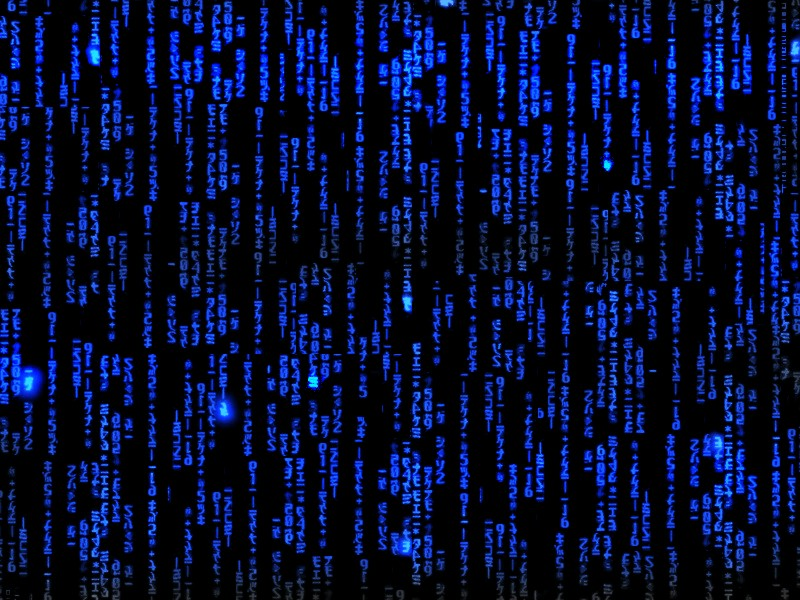Pure Programmer## Project: LCG Pseudo-Random Number Generator

The [[Linear congruential generator]] (LCG) [[pseudorandom number generator]] is the simplest and most studied way to generate a series of numbers that appear random.

The generator is defined by recurrence relation:

X_(n+1) = (aX_n+c) mod m

where X_0 is the seed or starting value and a, c and m define the period and behavior of the generator. A bad choice of a, c or m results in poorly generated values that repeat too frequently or correlate in other ways.

Write a LCG function to generate pseudorandom numbers. It should maintain an internal state X_n as a 64-bit integer but return the low 31-bits as the result of the generator. The function should accept the multiplier (a), increment (c) and modulus (m) as arguments. Start with X_0 as 3261963.

Write a program to test the function. The program should accept the multiplier (a), increment (c), modulus (m) and number of pseudorandom numbers to generate as arguments on the command line. Each time you call the generator print the raw value in hexadecimal and the floating point fraction in the range [0,1) computed using the raw value divided by 2^31

Notice that over multiple runs with the same a, c, and m, you will get the same results. This is because the seed is always the same. How could we get a seed that would be different each run?

See [[Linear_congruential_generator#Parameters_in_common_use|Common LCG Parameters]] for some choices to use when testing the LCG.

Output
$g++ -std=c++17 LCGPseudorandomGenerator.cpp -o LCGPseudorandomGenerator -lfmt$ ./LCGPseudorandomGenerator 19660D 3C6EF35F 100000000 20 multiplier: 1664525 increment: 1013904223 modulus: 4294967296 count: 32 6af163ee => 0.835492 1abfda75 => 0.208980 5ea4a950 => 0.739400 2f116c6f => 0.367719 285caf02 => 0.315328 4e10a279 => 0.609883 31d46984 => 0.389295 3426e713 => 0.407437 73554156 => 0.901039 713188bd => 0.884324 12e332f8 => 0.147559 327f59f7 => 0.394511 53ddeeea => 0.655210 40bf5141 => 0.505839 2fdef9ac => 0.373992 6408291b => 0.781499 14dccbbe => 0.162988 2a610005 => 0.331085 09daf1a0 => 0.076994 1172f87f => 0.136321 32af2bd2 => 0.395971 624bd909 => 0.767940 24a38ed4 => 0.286241 3659ac23 => 0.424612 08fda326 => 0.070240 2f07604d => 0.367413 77b28548 => 0.935136 75a26807 => 0.919019 13d005ba => 0.154786 2f7159d1 => 0.370647 1462c8fc => 0.159265 4223902b => 0.516710

Solution